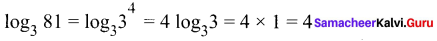# Samacheer Kalvi 11th Maths Solutions Chapter 2 Basic Algebra Ex 2.13

## Tamilnadu Samacheer Kalvi 11th Maths Solutions Chapter 2 Basic Algebra Ex 2.13

Choose the correct or the most suitable questions.

Question 1.
If |x + 2| ≤ 9, then x belongs to
(a) (-∞, -7)
(b) [-11, 7]
(c) (-∞, -7) ∪ [11, ∞)
(d)(-11, 7)
Solution:
(b) [-11, 7]
Hint:
-x – 2 ≤ 9 x + 2 ≤ 9
-x < 9 + 2 = 11 x ≤ 9 – 2 = 7
⇒ x ≥ -11
so x ∈ [-11, 7]

Question 2.
Given that x, y and b are real numbers x < y, b ≥ 0, then ……..
(a) xb < yb (b) xb > yb
(c) xb ≤ vb
(d) xlb ≥ ylb
Solution:
(a) xb < yb
Hint: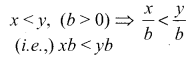Question 3.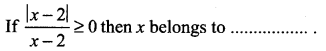(a) [2, ∞]
(b) (2, ∞)
(c) (-∞, 2)
(d) (-2, ∞)
Solution:
(b) (2, ∞)
Hint: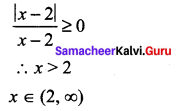Question 4.
The solution of 5x – 1 < 24 and 5x + 1 > -24 is …….
(a) (4, 5)
(b) (-5, -4)
(c) (-5, 5)
(d) (-5, 4)
Solution:
(c) (-5, 5)
Hint: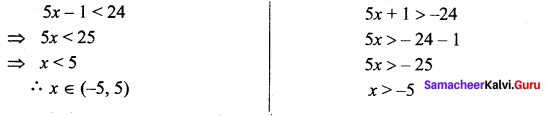Question 5.
The solution set of the following inequality |x – 1| ≥ |x – 3| is …….
(a) [0, 2]
(b) (2, ∞)
(c) (0, 2)
(d) (-∞, 2)
Solution:
(b) (2, ∞)

Question 6.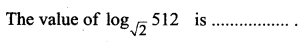(a) 16
(b) 18
(c) 9
(d) 12
Solution:
(b) 18
Hint: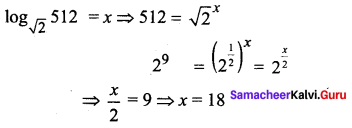Question 7.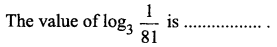(a) -2
(b) -8
(c) -4
(d) -9
Solution:
(c) -4
Hint: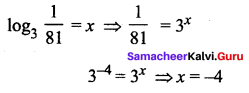Question 8.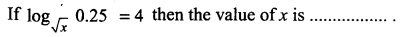(a) 0.5
(b) 2.5
(c) 1.5
(d) 1.25
Solution:
(a) 0.5
Hint: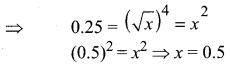Question 9.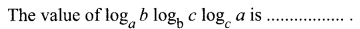(a) 2
(b) 1
(c) 3
(d) 4
Solution:
(b) 1
Hint: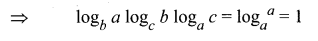Question 10.
If 3 is the logarithm of 343, then the base is ……
(a) 5
(b) 7
(c) 6
(d) 9
Solution:
(b) 7
Hint.
⇒ logx343 = 3 ⇒ 343 = x3
(.i.e.,) 73 = x3 ⇒ x = 7
⇒ x = 7

Question 11.
Find a so that the sum and product of the roots of the equation 2x2 + (a – 3)x + 3a – 5 = 0 are equal is ……..
(a) 1
(b) 2
(c) 0
(d) 4
Solution:
(b) 2
Hint: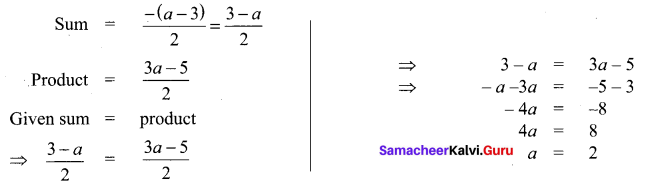Question 12.
If a and b are the roots of the equation x2 – kx + 16 = 0 and satisfy a2 + b2 = 32, then the value of k is ……
(a) 10
(b) -8
(c) (-8, 8)
(d) 6
Solution:
(c) -8, 8
Hint:
a + b = k ….(1) ab = 16 ….(2)
a2 + b2 = (a + b)2 – 2ab = 32 .
k2 – 32 = 32 ⇒ k2 = 64 ⇒ k = ±8

Question 13.
The number of solutions of x2 + |x – 1| = 1 is ………
(a) 1
(b) 0
(c) 2
(d) 3
Solution:
(c) 2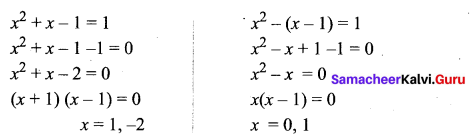We have two solutions 0, 1

Question 14.
The equations whose roots are numerically equal but opposite in sign to the roots of 3x2 – 5x – 7 = 0 is ……
(a) 3x2 – 5x – 7 = 0
(b) 3x2 + 5x – 7 = 0
(c) 3x2 – 5x + 7 = 0
(d) 3x2 + x – 7 = 0
Solution:
(b) 3x2 + 5x – 7 = 0
Hint: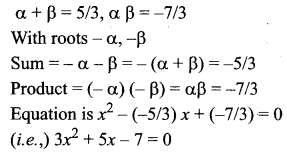Question 15.
If 8 and 2 are the roots of x2 + ax + c = 0 and 3, 3 are the roots of x2 + ax + b = 0, then the roots of the equation x2 + ax + b = 0 are …….
(a) 1, 2
(b) -1, 1
(c) 9, 1
(d) -1, 2
Solution:
(c) 9, 1
Hint:
Sum = 8 + 2 = 10 = -a ⇒ a = -10
Product = 3 × 3 = 9 = b ⇒ b = 9
Now the equation x2 + ax + b = 0
⇒ x2 – 10x + 9 = 0
⇒ (x- 9) (x – 1) = 0
x = 1 or 9

Question 16.
If a and b are the real roots of the equation x2 – kx + c = 0, then the distance
between the points (a, 0) and (b, 0) is ……..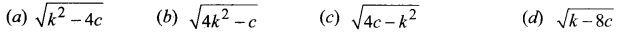Solution: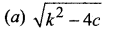Hint:
a + b = k, ab = c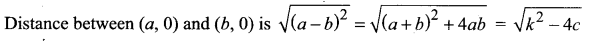Question 17.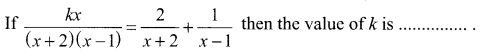(a) 1
(b) 2
(c) 3
(d) 4
Solution:
(c) 3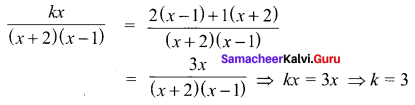Question 18.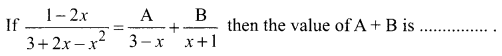(a) -1/2
(b) -2/3
(c) 1/2
(d) 2/3
Solution:
(a) -1/2
Hint: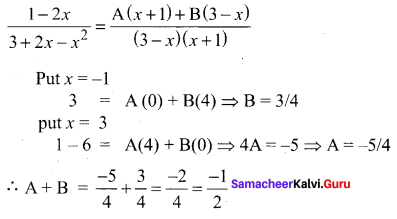Question 19.
The number of real roots of (x + 3)4 + (x + 5)4 = 16 is ……
(a) 4
(b) 2
(c) 3
(d) 0
Solution:
(a) 4
Hint:
The equation is (x + 3)4 + (x + 5)4 = 16
(x + 3)4 + (x + 5)4 = 24
This is biquadratic equation. It has 4 roots.

Question 20.
The value of log3 11 . log11 13 . log13 15 . log15 27 . log27 81 is …….
(a) 1
(b) 2
(c) 3
(d) 4
Solution:
(d) 4
Solution:
(d) 4
Hint.